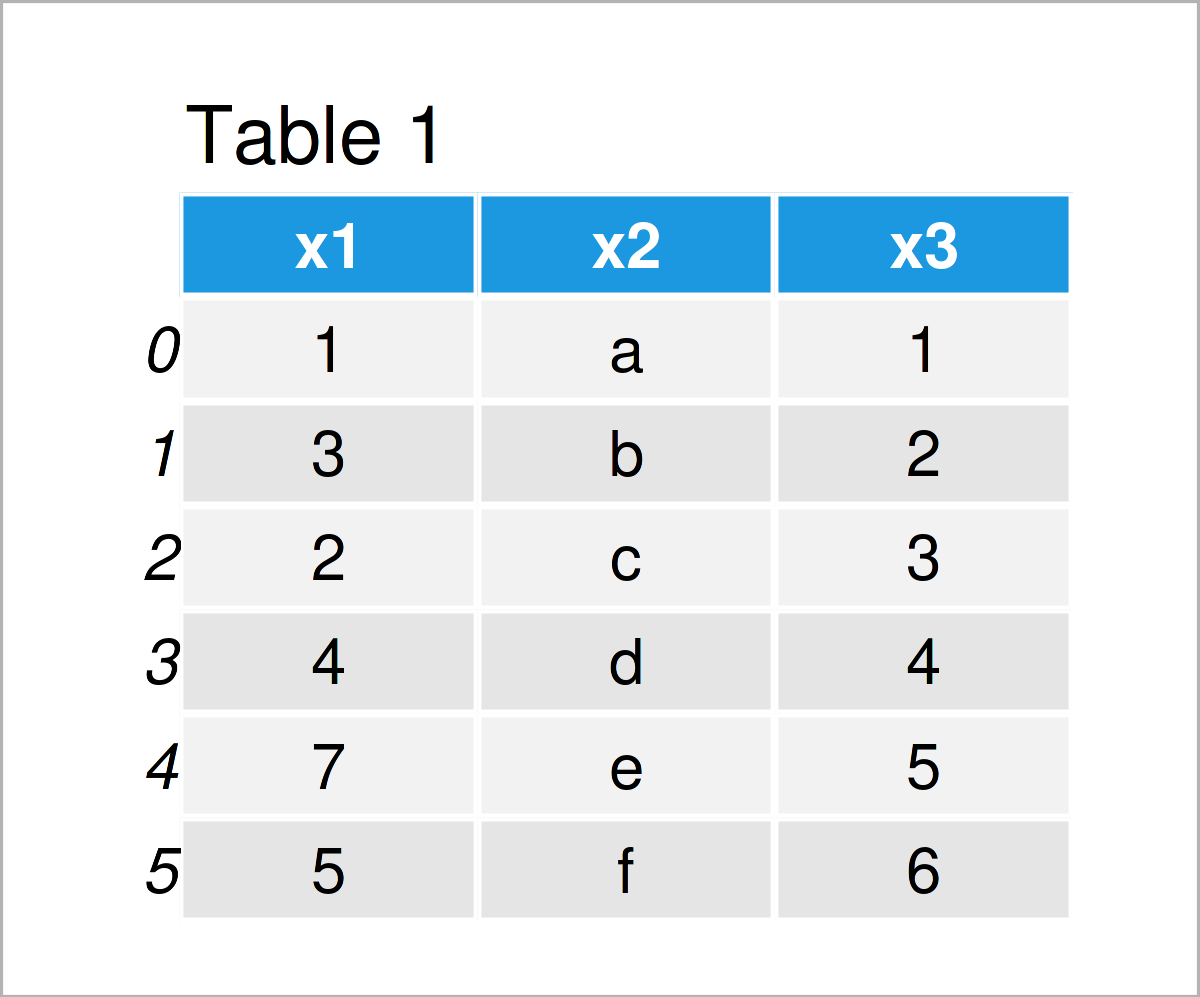# Find Differences Between Two Columns of pandas DataFrame in Python (3 Examples)

In this Python tutorial you’ll learn how to compare two columns of a pandas DataFrame.

The tutorial will consist of three examples for the comparison of two columns of a pandas DataFrame. To be more specific, the content of the article looks as follows:

Here’s how to do it…

## Example Data & Add-On Libraries

We first have to load the pandas library:

`import pandas as pd                              # Load pandas`

Next, we’ll also need to construct some example data.

```data = pd.DataFrame({'x1':[1, 3, 2, 4, 7, 5],    # Create pandas DataFrame
'x2':['a', 'b', 'c', 'd', 'e', 'f'],
'x3':range(1, 7)})
print(data)                                      # Print pandas DataFrame```Table 1 reveals the structure of our exemplifying data: It is a pandas DataFrame constructed of six rows and three columns.

The two columns x1 and x3 look similar, so let’s compare them in Python!

## Example 1: Check If All Elements in Two pandas DataFrame Columns are Equal

In Example 1, I’ll illustrate how to test whether each element of a first column is equal to each element of a second column.

For this task, we can use the equals function as shown below:

```print(data['x1'].equals(data['x3']))             # Apply equals function
# False```

After executing the previous Python code, the logical indicator False is returned, i.e. the columns x1 and x3 are not identical.

## Example 2: Check which Elements in Two pandas DataFrame Columns are Equal

As shown in Example 1, there are differences between the columns x1 and x3. Let’s check if at least some of the elements in these columns are the same.

To achieve this, we can use the == operator as shown in the following Python syntax:

```print(data['x1'] == data['x3'])                  # Apply logical operator
# 0     True
# 1    False
# 2    False
# 3     True
# 4    False
# 5    False
# dtype: bool```

The result of the previous code are multiple logical indicators that correspond to each row of our pandas DataFrame.

In case a logical operator is True, toe values in the columns x1 and x3 in this row are equal.

Note that this method takes the ordering of the values in our pandas DataFrame rows into account.

## Example 3: Check which Elements in First pandas DataFrame Column are Contained in Second

In this example, I’ll show how to check which of the values in a pandas DataFrame column are also contained in another column – no matter in which order the values are appearing.

To find this out, we can use the isin function as shown below:

```print(data['x1'].isin(data['x3']))               # Apply isin function
# 0     True
# 1     True
# 2     True
# 3     True
# 4    False
# 5     True
# Name: x1, dtype: bool```

Once again, we have returned several logical indicators. As you can see, only the value in the column x1 at the index position 4 is not contained in the variable x3.

## Video & Further Resources

Have a look at the following video on my YouTube channel. In the video, I’m explaining the examples of this page.

Furthermore, you might want to read some of the related articles on my website. I have released numerous other tutorials already.

To summarize: This page has demonstrated how to compare two columns of a pandas DataFrame and find differences in the Python programming language. In case you have further questions, please let me know in the comments.

Subscribe to the Statistics Globe Newsletter

•Aravind Viswanathan
October 10, 2022 9:10 am

Your resource is very helpful. I have a question on the example 3.
How do i right a condition with .isin and print a statement if all line items match.
Else, print a statement : At this line item the data does not match.

•November 14, 2022 11:57 am

Hey Aravind,

Thank you for the kind comment, glad you find the tutorials on Statistics Globe useful!

Please excuse the late response. I was on a long holiday so unfortunately I wasn’t able to reply sooner. Still need help with your code?

Regards,
Joachim

•Aravind Viswanathan
November 30, 2022 4:46 am

No Joachim,

I was able to solve it.

•